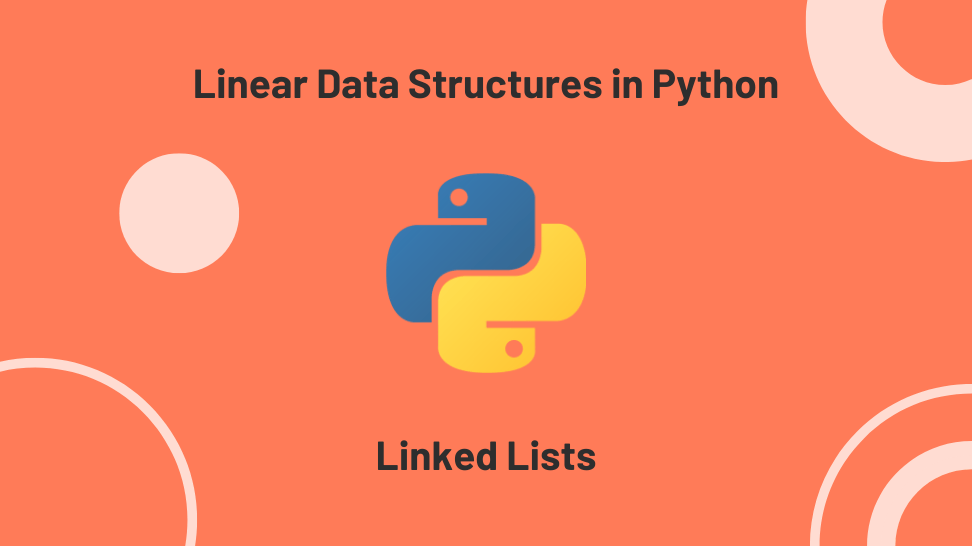In this article, I’ll explore the linked lists, a linear data structure we can easily implement in Python.

This post is the fourth in a miniseries exploring the implementation of linear data structures. I based the series on my notes while studying for a Python technical interview.

For quick access, here is the list of posts on this series:

1. A Stack Implementation in Python
2. A Queue Implementation in Python
3. A Priority Queue Implementation in Python

In computer science, a linked list is a linear collection of nodes whose order doesn’t depend on their physical placement in memory. Instead, each node contains the data and a reference (i.e., pointer) to the location of the next node in the collection.

The main benefit of linked lists over regular lists in Python is that you can easily insert or remove nodes without reallocating or reorganizing the entire data structure since nodes in linked lists are not stored contiguously.

1. Singly-Link Lists: where each node contains the data and a reference to the next node in the collection.
2. Doubly-Link Lists: where each node contains the data and references to the previous and next nodes in the collection.

In computer science, we sometimes use linked lists as a base while implementing other data structures like stacks and queues. Other uses include polynomials manipulation, arithmetic operations on long integers, and graph adjacency list representations.

You can also use linked lists for implementing the previous/next buttons in web browsers, music players, and image viewers.

In Python, the deque (double-ended-queue) from collections is a linked list implementation that comes with quite a few built-in methods and allows access, insertion, and removal from either end of the structure in constant time O(1).

For production code requiring a linked list, you should use this implementation.

Most linked lists implementation include the following methods:

1. ll.is_empty(): returns True is the linked list is empty.
4. ll.add_after(node_data, data): adds a node with data after the first occurrence of a node containing node_data.
5. ll.add_before(node_data, data): adds a node with data before the first occurrence of a node containing node_data.
6. ll.pop(): returns and removes the node at the front of the linked list.
7. ll.remove(node_data): removes the first occurrence of a node containing node_data.
8. ll.size(): returns the size of the linked list.

Since a linked list is a collection of nodes, we will need a Node class and a SinglyLinkedList class for our implementation.

### The Node class

The Node class has two attributes: a data attribute that accepts any data type and a next_node attribute that accepts a node object.

Below is our implementation.

We defined the SinglyLinkedList class with a constructor that sets the head of the list to None and a __str__ method that returns a readable representation of the linked list.

Since linked list nodes are not stored in contiguous memory spaces, you’ll need to traverse the list to find the correct location to perform certain operations. In our implementation, we added three helper methods that traverse the list:

1. sll.get _last_node(): returns the last node in the linked list.
2. sll.get_node(node_data): returns the first node that contains node_data.
3. sll.get_prev_node(node_data): returns the node before the first node that contains node_data.

Below is the implementation of all three methods. Since these methods potentially iterate over all nodes, they have a linear time complexity of O(n), where n is the length of the linked list.

### sll.is_empty():

The is_empty method returns True if the linked list is empty.

This method has a constant time complexity O(1).

This method has a constant time complexity O(1).

This method has a linear time complexity O(n), where n is the length of the linked list.

The add_after method adds a node with new_data after the first occurrence of a node with node_data in the linked list.

This method has a linear time complexity O(n), where n is the length of the linked list.

The add_before method adds a node with new_data before the first occurrence of a node with node_data in the linked list.

This method has a linear time complexity O(n), where n is the length of the linked list.

### sll.pop():

The pop method returns and removes the node at the front of the linked list.

This method has a constant time complexity O(1).

## sll.remove(node_data):

The remove method removes the node with the first occurrence node_data.

This method has a linear time complexity O(n), where n is the length of the linked list.

### sll.size():

The size method returns the number of nodes in the linked list.

This method has a linear time complexity O(n), where n is the length of the linked list.

### The full implementation

Below is the full implementation and some test cases:

Our implementation for a doubly-linked list follows. It only differs from the singly-linked list implementation in a few details:

1. The Node class has an attribute for the prev_nod, in addition to data and next_node.
2. The helper method get_prev_node in the DoublyLinkList class is no longer needed since we can now traverse forward and backward.
3. All the methods that mutate the linked list must update the relevant references to prev_node.

Here’s the full implementation and some test cases:

## Conclusion

This article covered linked lists, a linear data structure consisting of a collection of nodes. We reviewed their properties and uses and the methods included in typical implementations.

We finished by presenting implementations for a singly-linked list and a doubly-linked list.